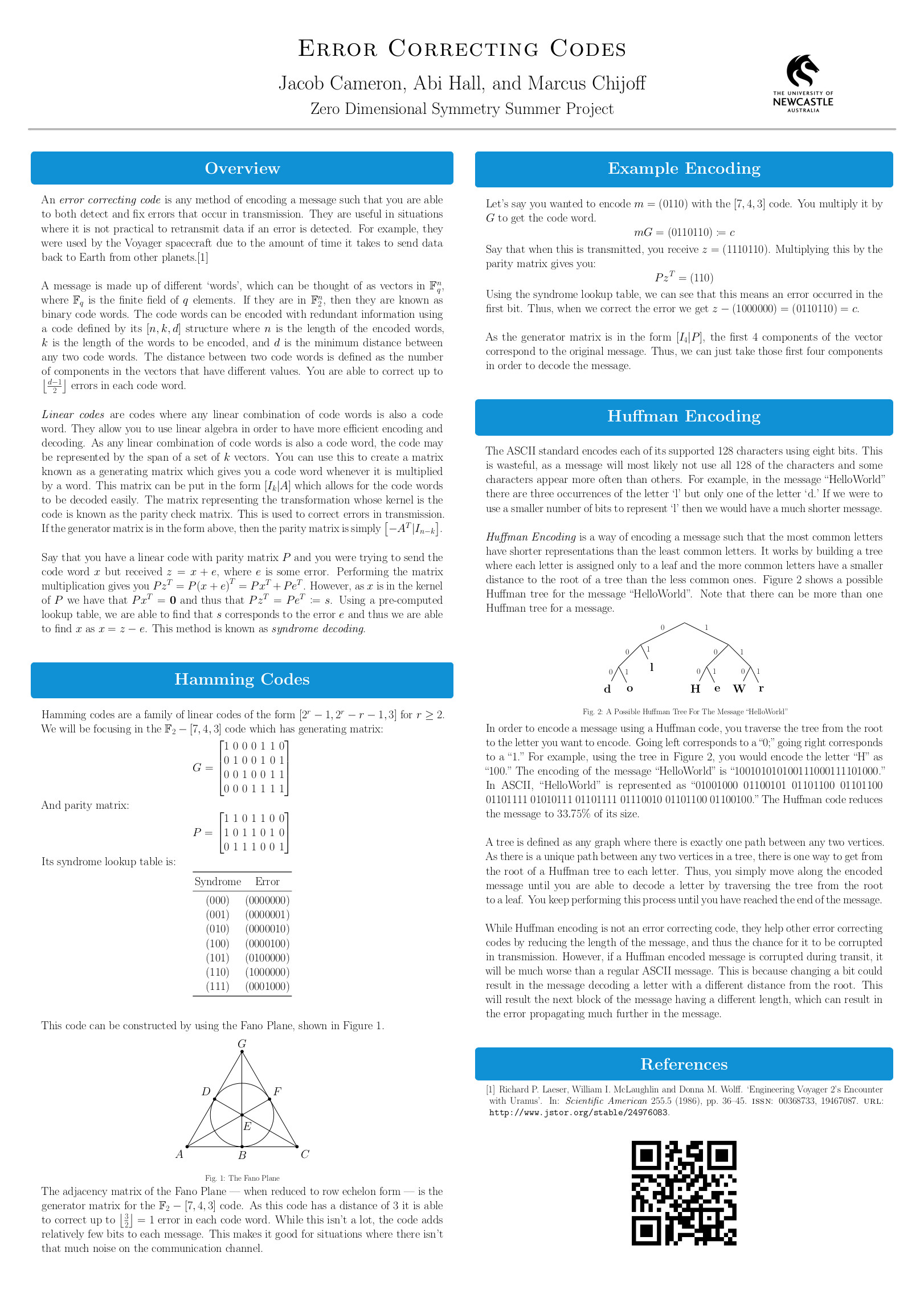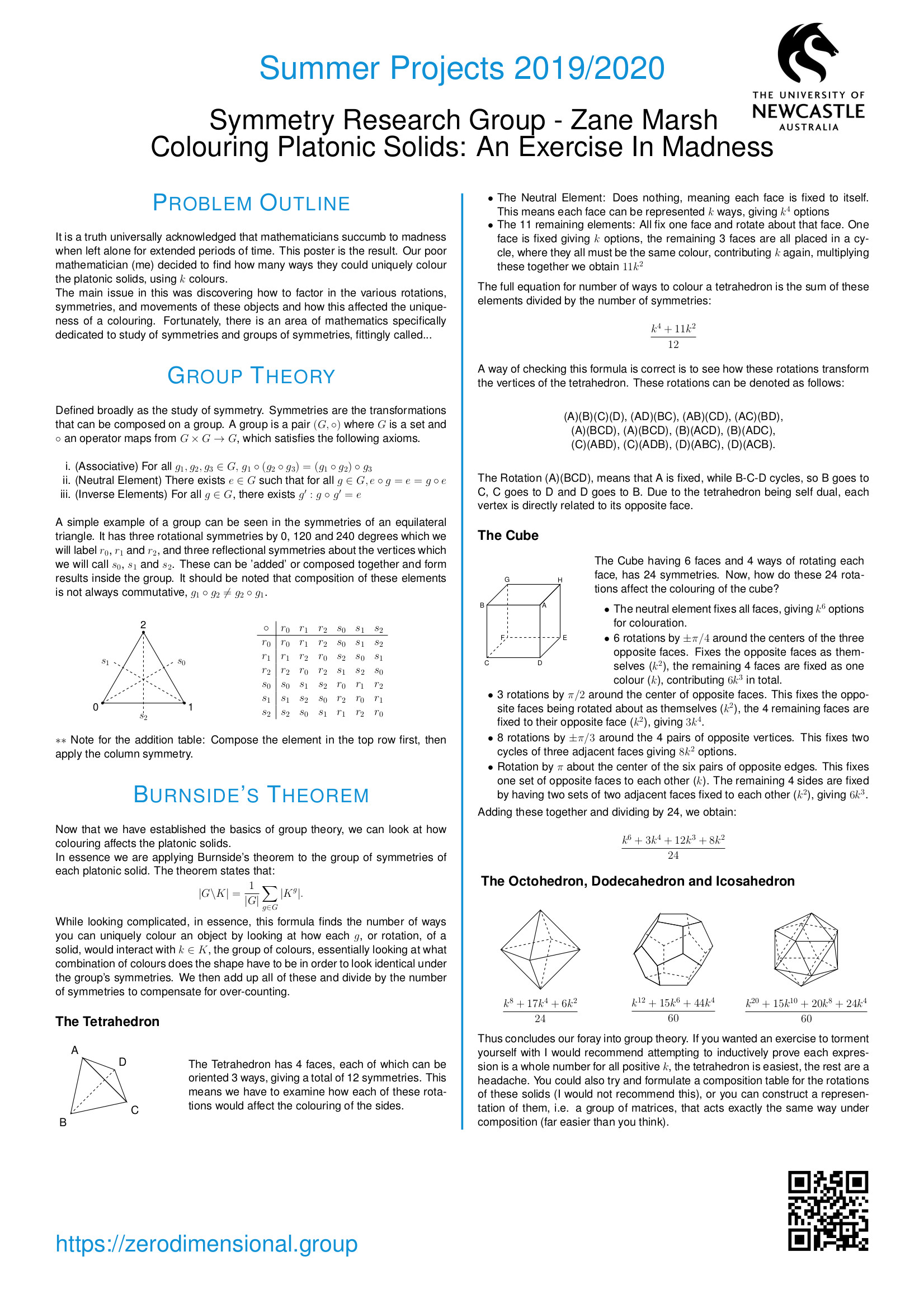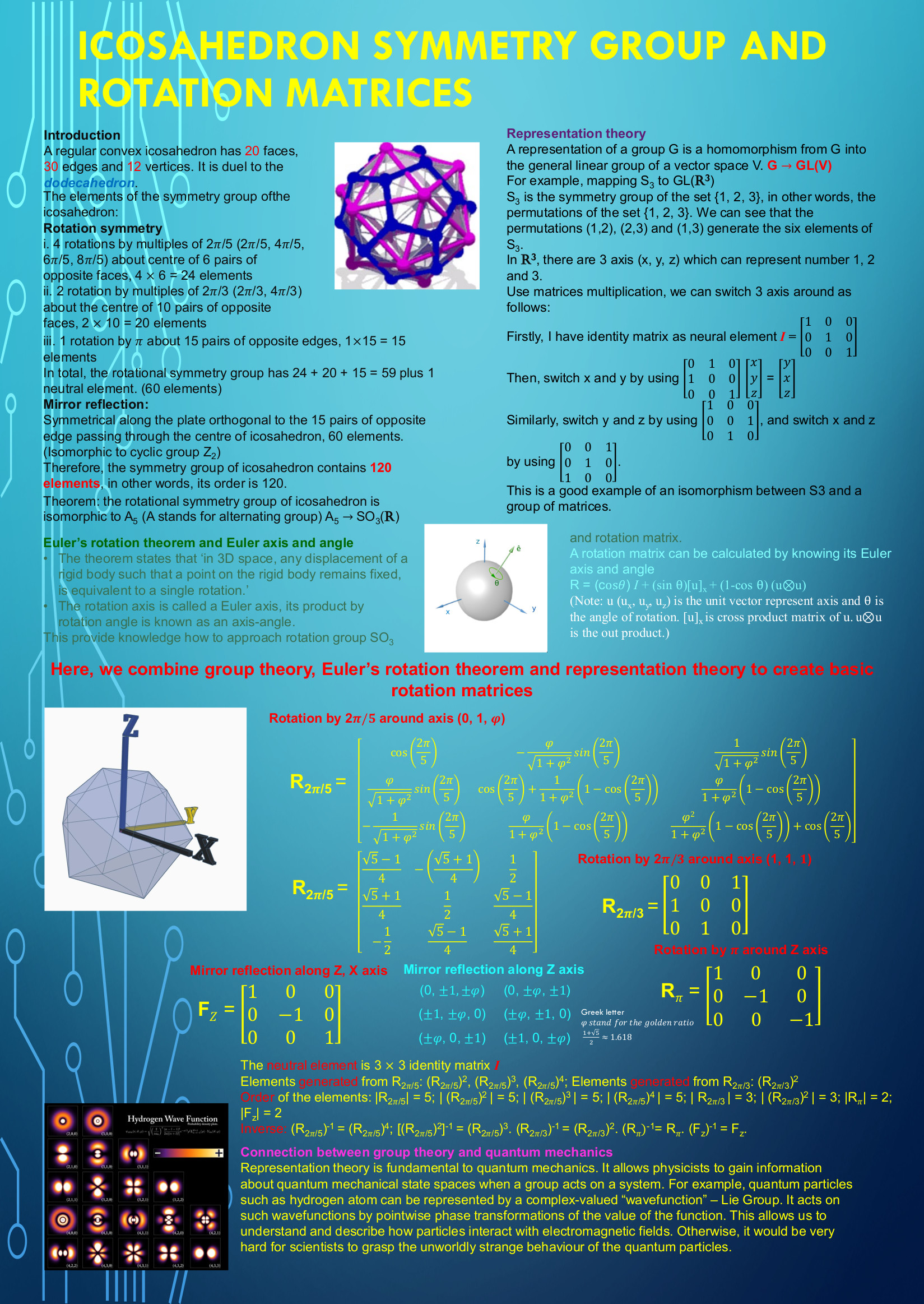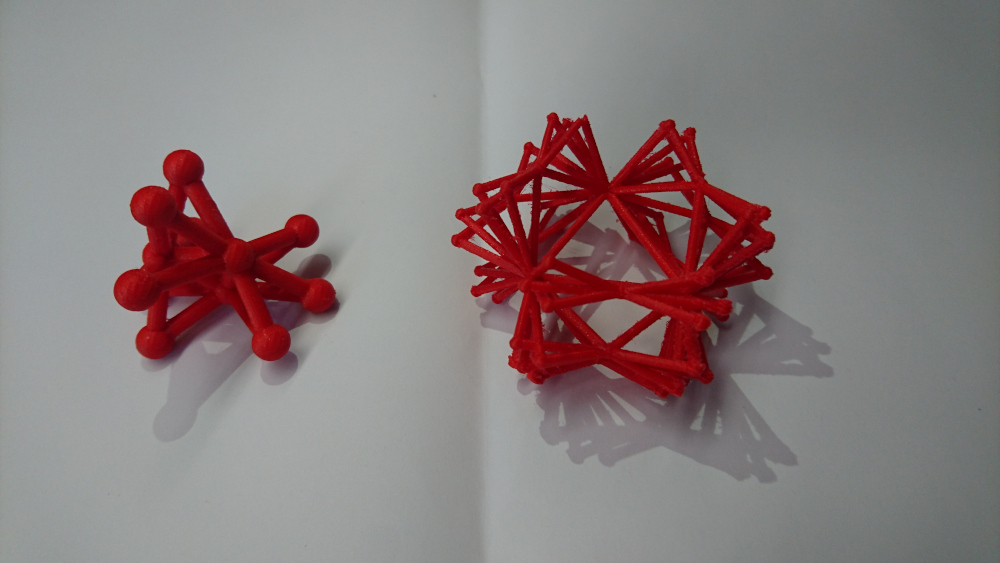# Current and Past Projects

### November 2022 - Februar 2023

Supervisor: Michal Ferov

Development and description of a paradigm for computing with automorphism groups of trees and analysis of approximations made in such computations.

## Writing a GAP package for local action diagrams

### Marcus Chijoff

Supervisors: Colin Reid, Stephan Tornier

By a result due to Colin Reid and Simon Smith, (P)-closed groups acting on a given infinite tree can be classified using "local action diagrams". A local action diagram is a finite connected graph whose vertices are labelled by permutation groups and whose arcs are labelled by sets relating to the orbits of the permutation groups that label the vertices. Properties of the group are reflected in its local action diagram and conversely. The goal of this project is to implement a category for local action diagrams in GAP and provide methods to create local action diagrams as well as test for relevant properties of them.

### Cameron Murtagh

Supervisor: George Willis

Self-replicating trees: Analysis and extension of a database, formulation and testing of conjectures suggested by the data, and creation of search tools for users of the database.

## Creating and analysing a library of universal groups

### Kenjie Balucan

Supervisor: Stephan Tornier

Mathematicicans study the intuitive notion of 'symmetry' through the algebraic notion of a 'group'. The symmetry 'groups' of infinite networks, or graphs, constitute a current research frontier and the 'universal groups' appearing in the title form an important class of examples. An existing computational package written in GAP provides methods to find and store universal groups. The goal of this project is to exhaust the capabilities of this package by running its methods on the university's high performance computing grid, organise the results into a library and, time permitting, analyse and visualise this library. Programming experience and basic knowledge of graph theory, combinatorics or linear algebra are essential. Knowledge of algebra (group theory) is desirable.

## Joining dots to see groups from trees

### Josiah Murray

Supervisor: George Willis

The project involves finding alternative ways of visualising self-similar permutation groups that improve our ability to see patterns among them. Several thousand of these groups, which act on binary trees, have been generated by computer searches. However, the action on the tree is often not illuminating and we seek geometric actions that convey more visual information. For example, given certain permutations of four points, our understanding of the permutations is greatly enhanced if we see that the four points are in fact the vertices of a square and the permutations come from rotations and reflections of the square. Our goal is to `join the dots’ and see geometric patterns in the computer generated cases we have found currently. The approach will again use a computer search. Knowledge of a second-year linear algebra or third-year algebra course is assumed, and knowledge of computing is desirable. Students will learn more algebra and also gain experience working with computer algebra systems. It is possible that work from this project will eventually be included in publicly available computer algebra packages. The project contributes to a much larger one that aims to understand symmetry groups of infinite networks, and students will also learn about this larger project.

## $k$-local actions of $\mathrm{PGL}(2,\mathbb{Q}_{p})$ acting on its Bruhat-Tits tree

### Tasman Fell

Supervisors: Michal Ferov, Stephan Tornier

The projective linear group over a local field has a transitive action on a highly symmetric combinatorial structure known as Bruhat-Tits building. In the case of 2-dimensional projective linear groups, the Bruhat-Tits building is know to be a regular tree. An automorphism of a tree can be fully described by its 'expansion' in terms of local actions. The aim of this project is to develop an algorithm that will, given an invertible matrix over the p-adic rationals, produce the local action expansion of the corresponding automorphism of the regular tree. The second part of this project consists of integrating this algorithm into the existing GAP package UGALY in order to generate the finite permutation groups that a vertex stabiliser in $\mathrm{PGL}(2,\mathbb{Q}_{p})$ induces on the sphere of a given radius around that vertex. Time permitting, we will tackle the same task for $\mathbb{F}_{p}[[t]]$ instead of $\mathbb{Q}_{p}$.

## Isolated points in colourings of vertex transitive graphs

### Max Carter

Supervisor: Michal Ferov

The aim of this project is to describe infinite symmetric graphs that admit a rigid colouring. In the case of locally finite graph, the space of all minimal colourings can be naturally seen as a topological space. This project is concerted with the question of to what extent does the topology of the space of colourings correspond to the geometry and symmetries of the graphs. First natural step is to study how far is the space of colourings away from being perfect, i.e. studying the isolated points. In the space of colourings, isolated points correspond to colourings that are rigid, meaning that if any other colouring agrees with it on a large enough finite subgraph, then they have to be the same. The goal of the project is to give some combinatorial of graphs that graphs that admit rigid colourings, or the converse - a combinatorial description of graphs that do not admit rigid colourings. The project is part of a program of research on 0-dimensional groups, which includes symmetries of infinite graphs.

## Local action expansions of $\mathrm{PGL}(2,\mathbb{Q}_{p})$ acting on its Bruhat-Tits tree

### Tasman Fell

Supervisors: Michal Ferov, George Willis

The projective linear group over a local field has a transitive action on a highly symmetric combinatorial structure known as Bruhat-Tits building. In the case of 2-dimensional projective linear groups, the Bruhat-Tits building is know to be a regular tree. An automorphism of a tree can be fully described by its 'expansion' in terms of local actions. The aim of this project is to develop an algorithm that will, given an invertible matrix over the p-adic rationals, produce the local action expansion of the corresponding automorphism of the regular tree. The second part of the project is to provide a partial inverse, i.e. an algorithm that will, provided a local action expansion of an automorphism of a regular tree of valency $p+1$, determine to which extent this automorphism can be approximated by a matrix over the p-adic rationals.

## Work Integrated Learning (COMP3851A): Groups acting on trees: compatible local actions

### Khalil Hannouch

Superviso: Stephan Tornier

The concept of symmetry is pervasive in mathematics and formalised in the algebraic notion of a 'group'. It is often natural to equip a group with a 'topology' - a generalisation of distance functions - which, in a sense, gives groups a shape. For example, the symmetry groups of infinite networks, or 'graphs', become zero-dimensional. A versatile and accessible class of these groups was defined by Burger-Mozes and refined by this project's supervisor: Picture an infinite graph in which every vertex has the same number of neighbours and consider only those symmetries of the graph which in a neighbourhood of every given vertex act like one of finitely many allowed 'local actions'. In order for the resulting family of symmetries to reflect these restrictions accurately, the local actions need to satisfy a certain compatibility condition. The aim of this project is to find new examples of local actions that satisfy the compatibility condition and to implement routines pertaining to these local actions in GAP.
This project has resulted in a GAP package which is available on github.

## Summer Project: Puzzles, Codes and Groups

### Jacob Cameron, Marcus Chijoff, Abigail Hall, Zane Marsh, Ellen Wu

Supervisors: Michal Ferov, Colin Reid, Stephan Tornier, George Willis

In this summer project, the above first-year students explored the mathematical formalisation of the everyday notion of symmetry, which is the algebraic concept of a 'group'. Using groups, we are able to state with certainty whether a given puzzle can be solved (the 15-puzzle can not!) and, if so, compute how many steps are needed. As an example of the far-reaching applicability of this concept, we look into error-correcting codes, such as the Golay code, which are critical in any digital communication, including NASA's Voyager program. Apart from theoretical studies in the area of groups, projects included the design, analysis and implementation of codes.## AMSI VRS Summer Project: Groups acting on trees without involutive inversions

### Jack Berry

Supervisor: Stephan Tornier

The concept of symmetry is pervasive in mathematics and formalised in the algebraic notion of a 'group'. It is often natural to equip a group with a 'topology' - a generalisation of distance functions - which, in a sense, gives groups a shape. For example, the symmetry groups of infinite networks, or 'graphs', become zero-dimensional. A versatile and accessible class of these groups was defined by Burger-Mozes and refined by this project's supervisor: Picture an infinite graph in which every vertex has the same number of neighbours and consider only those symmetries of the graph which in a neighbourhood of every given vertex act like one of finitely many allowed 'local actions'. In order for the resulting family of symmetries to reflect these restrictions accurately, the local actions need to satisfy certain conditions. The aim of this project is to define a new class of groups acting on said graph by restricting the local action on edge neighbourhoods rather than vertex neighbourhoods, and thereby gain a new perspective on existing examples of graph symmetry groups relating to the Weiss conjecture.

## Project (SCIE3500): Decomposition theorems for automorphism groups of trees

### Max Carter

Supervisor: George Willis

We investigated double coset decompositions of automorphism groups of trees that preserve vertex labellings. The idea was to compare with the Bruhat and Cartan decompositions of simple Lie groups and to see whether they could be used to prove results about the groups in a similar way in which the Cartan and Bruhat decompositions are. We were in fact able to prove that every continuous homomorphism from the automorphism group of a label-regular tree has closed range. Moreover, the work suggested problems concerning abstract properties of double coset decompositions that are the subject of further research. An associated article is accepted for publication in the Bulletin of the Australian Mathematical Society.

## Winter Project: Visualisations of buildings

### Tasman Fell

Supervisor: Michal Ferov

Many groups can be understood by studying objects on which the group acts by symmetries. In fact, every compactly generated totally disconnected locally compact group acts transitively on a locally finite graph. In the case of linear groups over the p-adics these combinatorial objects are known as the Tits-buildings. In general, the construction of these graphs relies on the axiom of choice and as such cannot really be effectively implemented. However, in the case of linear groups over the p-adics, we can effectively approximate the p-adics by rational numbers and construct a finite proportion of the corresponding building. The aim of this project is to visualise finite propositions of buildings associated to to the special linear groups $\mathrm{SL}(2,\mathbb{Q}_2)$ and $\mathrm{SL}(3,\mathbb{Q}_p)$.## AMSI VRS Summer Project: Simple groups of infinite matrices

### Peter Groenhout

Supervisors: Colin Reid, George Willis

An essential step towards understanding $0$-dimensional symmetry is to describe the totally disconnected, locally compact (t.d.l.c.) groups which are simple. Simple groups are those which cannot be factored into smaller pieces and they are sometimes called the 'atoms of symmetry', or said to be analogues of the prime numbers in number theory. This project investigates t.d.l.c. groups of infinite matrices. It is suspected that these groups will be found to be simple and we will aim to show that by first studying corresponding groups of $n\times n$ matrices which are known to be simple. Students taking this project will extend their knowledge of algebra, analysis and number theory. 'Totally disconnected', 'locally compact' and '$0$-dimensional' are topological notions; 'group' and 'simple' are algebraic ones; and the matrix entries are numbers modulo a prime number $p$.
Continuing work on this project resulted in a preprint which can be found here.

## AMSI VRS Summer Project: Free products of graphs

### Max Carter

Supervisors: Stephan Tornier, George Willis

Symmetries of networks (or graphs) are '$0$-dimensional', and such symmetries are investigated through the algebraic technique of totally disconnected, locally compact groups. We are interested in highly symmetric, infinite graphs and one way to form such graphs is by gluing together infinitely many copies of finite graphs according to some regular instructions. This project investigates the symmetry groups of examples of graphs formed in this way and compares them with the symmetry groups of infinite regular trees, which are the most basic type of infinite regular graph. The aim is to determine whether the symmetry groups obtained in this way are simple and new. 'Totally disconnected' and 'locally compact' are topological notions; 'group' and 'simple' are algebraic ones; and 'graphs' are a combinatorial concept.
Continuing work on this project has resulted in a publication in the Australasian Journal of Combinatorics.

## AMSI VRS Summer Project: Random Walks on Derived Graphs

### Alastair Anderberg

Supervisor: Dave Robertson

A simple random walk through $\mathbb{Z}^{d}$ describes a path taken through a d-dimensional integer lattice, where each of the 2d possible directions is chosen with equal probability. In 1921, George Polya proved that $d=1$ or $d=2$ dimensions, such a path will return to its starting position almost surely, but for $d=3$ dimensions or higher, the probability of returning to the the starting position decreases as the number of dimensions increases. In this project, we plan to generalise this idea from the integer lattice to more complicated structures through the idea of derived graphs.